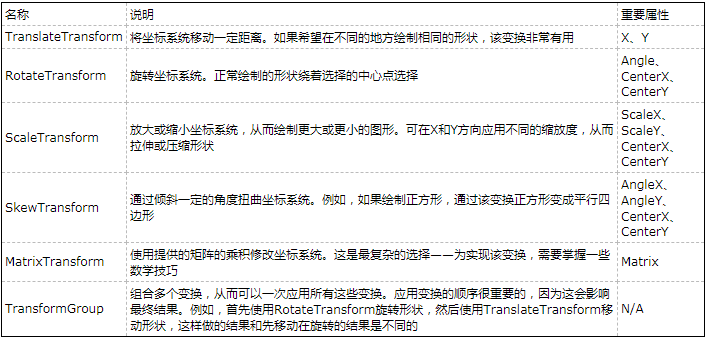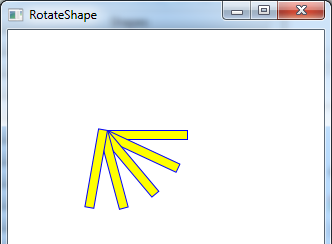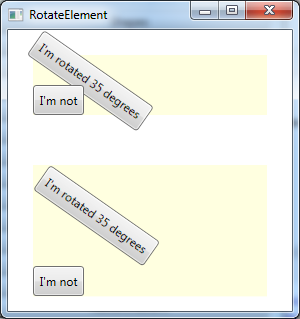# 【WPF学习】第四十一章 变换

2019/04/10 10:10

通过使用变换(transform)，许多绘图任务将更趋简单；变换是通过不加通告地切换形状或元素使用的坐标系统来改变形状或元素绘制方式的对象。在WPF中，变换由继承自System.Windows.Media.Transform抽象类的类表示。下表列出了这些类。从技术角度看，所有变换都使用矩阵数学改变形状的坐标。不过，使用预先构建好的变换，如TranslateTransform、RotateTransform、ScaleTransform以及SkewTransform，比使用MatrixTransform并尝试为希望执行的操作构造正确的矩阵更简单的多。当使用TransformGroup执行一系列变换时，WPF将所有变换融合到单独的MatrixTransform变换中以确保获得最佳性能。

所有变换(通过Transform类)继承自Freezable类，这意味着它们支持自动更改通知功能。如果改变了在形状中使用的变换，形状会立即重新绘制自身。

变换是那些在不同上下文中非常有用的古怪概念中的一个。下面例举几个例子：

• 　　倾斜形状。到目前为止已经介绍了水平对齐的矩形、椭圆、直线以及多边形。使用RotateTransform变换，可转动坐标系统，使创建特定的形状更容易。
• 　　重复形状。许多图画是在不同的位置使用类似的形状构建的。使用变换，可先绘制一个形状，然后移动、旋转、缩放该形状，以及执行其他操作。
• 　　动画。通过变换，可创建大量精致的效果。例如，旋转形状、将形状从一个地方移到另一个地方，以及动态扭曲形状。

为变换形状，将RenderTransform属性指定为希望使用的变换队形。根据使用的变换队形，需要填充不同的属性以配置变换队形。

例如，如果旋转形状，需要使用RotateTransform变换，并以度为单位提供旋转角度。下面的示例将举行旋转25°：

<Rectangle Width="80" Height="10" Stroke="Blue" Fill="Yellow"
Canvas.Left="100" Canvas.Top="100">
<Rectangle.RenderTransform>
<RotateTransform Angle="25" />
</Rectangle.RenderTransform>
</Rectangle>

采用这种方式旋转形状时，是围绕形状的原点进行旋转的（左上角）。下图演示了绕形状原点旋转25°、50°、75°以及100°的效果。<Window x:Class="Drawing.RotateShape"
xmlns="http://schemas.microsoft.com/winfx/2006/xaml/presentation"
xmlns:x="http://schemas.microsoft.com/winfx/2006/xaml"
Title="RotateShape" Height="427" Width="332"
>
<Canvas>
<Rectangle Width="80" Height="10" Stroke="Blue" Fill="Yellow"
Canvas.Left="100" Canvas.Top="100">
</Rectangle>

<Rectangle Width="80" Height="10" Stroke="Blue" Fill="Yellow"
Canvas.Left="100" Canvas.Top="100">
<Rectangle.RenderTransform>
<RotateTransform Angle="25" />
</Rectangle.RenderTransform>
</Rectangle>

<Rectangle Width="80" Height="10" Stroke="Blue" Fill="Yellow"
Canvas.Left="100" Canvas.Top="100">
<Rectangle.RenderTransform>
<RotateTransform Angle="50" />
</Rectangle.RenderTransform>
</Rectangle>

<Rectangle Width="80" Height="10" Stroke="Blue" Fill="Yellow"
Canvas.Left="100" Canvas.Top="100">
<Rectangle.RenderTransform>
<RotateTransform Angle="75" />
</Rectangle.RenderTransform>
</Rectangle>

<Rectangle Width="80" Height="10" Stroke="Blue" Fill="Yellow"
Canvas.Left="100" Canvas.Top="100">
<Rectangle.RenderTransform>
<RotateTransform Angle="100" />
</Rectangle.RenderTransform>
</Rectangle>
</Canvas>

</Window>
RotateShape有时候希望绕不同的点旋转形状。与其他许多变换类一样，RotateTransform变换也提供了CenterX和CentertY属性。可以用这些属性指定将进行旋转的中心。下面的矩形使用该方法绕其中心点旋转自身25°：

<Rectangle Width="80" Height="10" Stroke="Blue" Fill="Yellow"
Canvas.Left="100" Canvas.Top="300">
<Rectangle.RenderTransform>
<RotateTransform Angle="25" CenterX="45" CenterY="5" />
</Rectangle.RenderTransform>
</Rectangle>

采用这种方式旋转形状时，是围绕形状的原点进行旋转的（中心）。下图演示了绕形状原点旋转25°、50°、75°以及100°的效果。<Window x:Class="Drawing.RotateShape"
xmlns="http://schemas.microsoft.com/winfx/2006/xaml/presentation"
xmlns:x="http://schemas.microsoft.com/winfx/2006/xaml"
Title="RotateShape" Height="427" Width="332"
>
<Canvas>
<Rectangle Width="80" Height="10" Stroke="Blue" Fill="Yellow"
Canvas.Left="100" Canvas.Top="300">
</Rectangle>

<Rectangle Width="80" Height="10" Stroke="Blue" Fill="Yellow"
Canvas.Left="100" Canvas.Top="300">
<Rectangle.RenderTransform>
<RotateTransform Angle="25" CenterX="45" CenterY="5" />
</Rectangle.RenderTransform>
</Rectangle>

<Rectangle Width="80" Height="10" Stroke="Blue" Fill="Yellow"
Canvas.Left="100" Canvas.Top="300">
<Rectangle.RenderTransform>
<RotateTransform Angle="50" CenterX="45" CenterY="5" />
</Rectangle.RenderTransform>
</Rectangle>

<Rectangle Width="80" Height="10" Stroke="Blue" Fill="Yellow"
Canvas.Left="100" Canvas.Top="300">
<Rectangle.RenderTransform>
<RotateTransform Angle="75" CenterX="45" CenterY="5" />
</Rectangle.RenderTransform>
</Rectangle>

<Rectangle Width="80" Height="10" Stroke="Blue" Fill="Yellow"
Canvas.Left="100" Canvas.Top="300">
<Rectangle.RenderTransform>
<RotateTransform Angle="100" CenterX="45" CenterY="5" />
</Rectangle.RenderTransform>
</Rectangle>
</Canvas>

</Window>
RotateShape使用RotateTransform的CenterX和CenterY属性时存在明显的限制。这些属性是使用绝对坐标定义的，这意味着需要了解绘制内容的中心店的准确位置。如果正在显示动态内容（例如，可变维度的图片或改变尺寸的元素），就会出现问题。幸运的是，WPF通过方便的RenderTransformOrigin属性，为这个问题提供了解决方法，所以形状都支持RenderTransformOrign属性。该属性使用相对坐标系统设置中心点，下该对坐标系统在两个方向上的范围都是从0到1。换句话说，点（0,0）被指定为左上角，点（1,1）表示右下角（如果形状区域不是正方形，那么会相应地拉伸坐标系统）。

借助于RenderTransformOrigin属性，可使用如下所示的标记，绕中心点旋转任意形状：

<Rectangle Width="80" Height="10" Stroke="Blue" Fill="Yellow"
Canvas.Left="100" Canvas.Top="300" RenderTransformOrigin="0.5,0.5">
<Rectangle.RenderTransform>
<RotateTransform Angle="75" CenterX="45" CenterY="5" />
</Rectangle.RenderTransform>
</Rectangle>

因为不管形状的尺寸是多少，点（0.5,0.5）都表示形状中心，所以上面的标记可以工作。实际上，RenderTransformOrigin属性通常比CenterX和CenterY属性更有用，尽管根据需要可以使用两者中的一个，或者同时使用两者。

RenderTransform和RenderTransformOrigin属性并不限制只能用于形状。实际上，Shape类的这些属性从UIElement类继承而来，这意味着所有WPF元素都支持这两个属性，包括按钮、文本框、TextBlock控件、充满内容的整个布局容器等。令人感到惊讶的是，可旋转、扭曲以及缩放WPF用户界面中的任意一部分。

RenderTransform不是在WPF基类中定义的唯一与变换相关的属性。FrameworkElement类还定义了LayoutTransform属性。LayoutTransform属性以相同的方式变换元素，但在布局之前执行其工作。这种情况的开销虽然更大些，但如果使用布局容器为一组控件提供自动布局功能，这种方式是很关键的（Shape类也提供了LayoutTransform属性，但很少需要使用该属性，因此通常使用容器（如Canvas面板）明确地放置形状，而不是使用自动布局）。

为理解两者的区别，分析下图中显示的窗口，该窗口包含两个StackPanel容器（由阴影区域表示），这两个容器都包含一个选择过的按钮和一个正常的按钮。在第一个StackPanel容器中，选择的按钮使用RenderTransform方法。该StackPanel容器在对两个按钮进行布局时，第一个按钮正常定位，并且在即将呈现之前旋转该按钮。因此，选择过的按钮被重叠在下面。在第二个StackPanel容器中，选择过的按钮使用LayoutTransform方法。StackPanel容器获取到选项后按钮所需的边界，并相应地布局第二个按钮。<Window x:Class="Drawing.RotateElement"
xmlns="http://schemas.microsoft.com/winfx/2006/xaml/presentation"
xmlns:x="http://schemas.microsoft.com/winfx/2006/xaml"
Title="RotateElement" Height="314" Width="305"
>
<StackPanel>
<StackPanel  Margin="25"  Background="LightYellow">
<Button.RenderTransform>
<RotateTransform Angle="35" CenterX="45" CenterY="5" />
</Button.RenderTransform>
<Button.Content>I'm rotated 35 degrees</Button.Content>
</Button>
</StackPanel>

<StackPanel  Margin="25"  Background="LightYellow">
<Button.LayoutTransform>
<RotateTransform Angle="35" CenterX="45" CenterY="5" />
</Button.LayoutTransform>
<Button.Content>I'm rotated 35 degrees</Button.Content>
</Button>
</StackPanel>
</StackPanel>
</Window>
RotateElement只有很少几个元素不能被变换，因为他们的呈现工作并非由WPF本身负责。不能被变换的元素的两个例子是WindowsFormHost和WebBrower元素，WindowsFormHost元素用于在WPF窗口中放置Windows窗体控件，WebBrower元素用于显示HTML内容。

在一定程度上，当设置LayoutTransform或RenderTransform属性时，WPF元素不知道它们正在被修改。特别是，变换不会影响元素的ActualHeight和ActualWidth属性，它们仍记录着变换之前的值。这正是WPF能够保证流失布局以及外边距继续以相同的方式工作的部分原理，即使应用了一个或多个变换也同样如此。

0
0 收藏

### 作者的其它热门文章0 评论
0 收藏
0